# JP5553196B2 - Vehicle reflector - Google Patents

## Info

Publication number
JP5553196B2
JP5553196B2 JP2009193432A JP2009193432A JP5553196B2 JP 5553196 B2 JP5553196 B2 JP 5553196B2 JP 2009193432 A JP2009193432 A JP 2009193432A JP 2009193432 A JP2009193432 A JP 2009193432A JP 5553196 B2 JP5553196 B2 JP 5553196B2
Authority
JP
Japan
Prior art keywords
angle
inclination angle
degrees
rotation angle
reference axis
Prior art date
Legal status (The legal status is an assumption and is not a legal conclusion. Google has not performed a legal analysis and makes no representation as to the accuracy of the status listed.)
Active
Application number
JP2009193432A
Other languages
Japanese (ja)
Other versions
JP2011043760A (en
Inventor

Original Assignee
スタンレー電気株式会社
Priority date (The priority date is an assumption and is not a legal conclusion. Google has not performed a legal analysis and makes no representation as to the accuracy of the date listed.)
Filing date
Publication date
Application filed by スタンレー電気株式会社 filed Critical スタンレー電気株式会社
Priority to JP2009193432A priority Critical patent/JP5553196B2/en
Publication of JP2011043760A publication Critical patent/JP2011043760A/en
Application granted granted Critical
Publication of JP5553196B2 publication Critical patent/JP5553196B2/en
Application status is Active legal-status Critical
Anticipated expiration legal-status Critical

## Images

•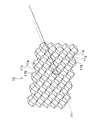•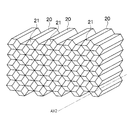•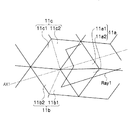•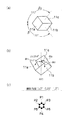•••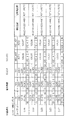•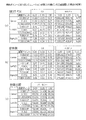•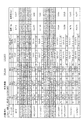•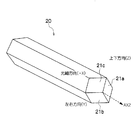•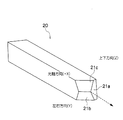•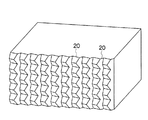•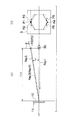•••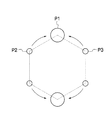## Classifications

• FMECHANICAL ENGINEERING; LIGHTING; HEATING; WEAPONS; BLASTING
• F21LIGHTING
• F21VFUNCTIONAL FEATURES OR DETAILS OF LIGHTING DEVICES OR SYSTEMS THEREOF; STRUCTURAL COMBINATIONS OF LIGHTING DEVICES WITH OTHER ARTICLES, NOT OTHERWISE PROVIDED FOR
• F21V7/00Reflectors for light sources
• F21V7/04Optical design
• GPHYSICS
• G02OPTICS
• G02BOPTICAL ELEMENTS, SYSTEMS, OR APPARATUS
• G02B5/00Optical elements other than lenses
• G02B5/12Reflex reflectors
• G02B5/122Reflex reflectors cube corner, trihedral or triple reflector type
• G02B5/124Reflex reflectors cube corner, trihedral or triple reflector type plural reflecting elements forming part of a unitary plate or sheet
• FMECHANICAL ENGINEERING; LIGHTING; HEATING; WEAPONS; BLASTING
• F21LIGHTING
• F21WINDEXING SCHEME ASSOCIATED WITH SUBCLASSES F21K, F21L, F21S and F21V, RELATING TO USES OR APPLICATIONS OF LIGHTING DEVICES OR SYSTEMS
• F21W2107/00Use or application of lighting devices on or in particular types of vehicles

## Description

The present invention relates to a vehicle reflector, and more particularly to a vehicle reflector capable of improving the light intensity at a measurement point where the light intensity in the observation angle direction is required.

Conventionally, in the field of vehicle reflectors, it has been required to increase the luminous intensity in the observation angle direction, and for example, the one described in Patent Document 1 has been proposed as a response to this requirement.

As shown in FIGS. 14 and 15, the vehicle reflector described in Patent Document 1 tilts incident light incident in parallel to a horizontally arranged reference axis AX by a predetermined angle with respect to the reference axis AX. A plurality of retroreflective elements 211 that retroreflect in the direction are included. The retroreflective element 211 includes a first reflecting surface 1, a second reflecting surface 2, and a third reflecting surface 3 that are adjacently arranged so as to form a substantially cubic corner (see FIG. 15). 1 to 3 are inclined at the same angle (about 35 ° 17 ′) with respect to the reference axis AX arranged in the horizontal direction (see FIG. 14), and the second reflecting surface 2 and the third reflecting surface 3 are the reference. It is formed at a position rotated about the axis AX by the same angle (± 120 ° 6 ′) (see FIG. 15).

In the vehicle reflector 210 configured as described above, incident light incident in parallel to the reference axis AX arranged in the horizontal direction is caused by the action of the retroreflective elements (first to third reflecting surfaces 1 to 3). Measurement that reflects obliquely upward by a predetermined angle with respect to the reference axis AX and, as shown in FIG. 16, the light collecting points P2 and P3 on both sides approach the uppermost light collecting point P1, and therefore the light intensity in the observation angle direction is required. It becomes possible to improve the luminous intensity of the point.

Japanese Patent No. 3340640

The inventor of the present application intensively studied to further improve the light intensity at the measurement point where the light intensity in the observation angle direction is required, and as a result, the first inclination angle (ANG1), the second inclination angle (ANG2), the third When the total five parameters of the tilt angle (ANG3), the downward rotation angle (ROT1), and the upward rotation angle (ROT2) satisfy the relationship of the following equation, the luminous intensity at the measurement point required by the standard of the reflex reflector is It has been found that it is possible to obtain a maximum luminous intensity higher than that described in Patent Document 1.

ANG2> ANG3 (Formula 1)
ANG2> ANG1 (Formula 2)
ROT1>ROT2> 120 degrees (Equation 3)
However, the first tilt angle (ANG1) is the tilt angle with respect to the reference axis of the first reflecting surface, the second tilt angle (ANG2) is the tilt angle with respect to the reference axis of the second reflecting surface, and the third tilt angle (ANG3) is the third angle. The inclination angle of the reflecting surface with respect to the reference axis and the downward rotation angle (ROT1) are the rotation angle of the second inclined surface around the reference axis from the reference surface (first reflection surface), and the upward rotation angle (ROT2) is This is the rotation angle of the third inclined surface around the reference axis from the reference surface (first reflection surface).

The present invention has been made based on the above knowledge, and can form a vehicle reflector and a vehicle reflector capable of further improving the light intensity at a measurement point where the light intensity in the observation angle direction is required. The aim is to provide a possible reflex pin.

To solve the above problems, a first aspect of the present invention, and retroreflected at a predetermined angle obliquely upward incident light that is incident parallel to the reference placed on the horizontal axis with respect to the reference axis predetermined In the vehicle reflector including a plurality of retroreflective elements configured to condense in the observation angle direction, the retroreflective element is a first reflective surface disposed adjacent to form a substantially cubic corner, A second reflective surface and a third reflective surface, wherein the first reflective surface is inclined at a first inclination angle with respect to the reference axis, and the second reflective surface is The third reflection surface is formed at a position inclined by two inclination angles and rotated by a first rotation angle about the reference axis from the first reflection surface, and the third reflection surface has a third inclination angle with respect to the reference axis. The second tilted and centered on the reference axis from the first reflecting surface. The first tilt angle, the second tilt angle, the third tilt angle, the first rotation angle, and the second rotation angle are represented by the following formulas. The vehicle reflector characterized by satisfy | filling. ANG2> ANG3, ANG2> ANG1, ROT1>ROT2> 120 degrees. However, ANG1: 1st inclination angle, ANG2: 2nd inclination angle, ANG3: 3rd inclination angle, ROT1: 1st rotation angle, ROT2: 2nd rotation angle.

According to the first aspect of the present invention, unlike the prior art, the first tilt angle, the second tilt angle, the first tilt angle, and so on so as to satisfy the angle conditions of ANG2> ANG3, ANG2> ANG1, ROT1> ROT2> 120 degrees. Since each of the five parameters of the three tilt angles, the first rotation angle, and the second rotation angle is set (the prior art only sets two parameters in practice), the luminous intensity in the observation angle direction is required. It becomes possible to further improve the luminous intensity of the measurement point as compared with the conventional case.

The invention according to claim 2 is the invention according to claim 1, wherein the predetermined observation angle is 0.33 degrees.

According to the second aspect of the present invention, the measurement point for which the luminous intensity in the observation angle (0.33 degree) direction is obtained by satisfying the angular conditions of ANG2> ANG3, ANG2> ANG1, ROT1> ROT2> 120 degrees. The luminous intensity can be improved as compared with the conventional case.

The invention according to claim 3 is the invention according to claim 2, wherein the first inclination angle is 35.273 degrees ± 0.0027 degrees, and the second inclination angle is 35.310 degrees ± 0.0027. The third tilt angle is 35.305 degrees ± 0.0027 degrees, the first rotation angle is 120.07 degrees ± 0.0027 degrees, and the second rotation angle is 120.06 degrees ± 0.00. It is characterized by being 0027 degrees.

According to the third aspect of the invention, the first inclination angle = 35.273 degrees ± 0.0027 degrees, the second inclination angle = 35.310 degrees ± 0.0027 degrees, and the third inclination angle = 35.305 degrees. Even if it is set within the range of ± 0.0027 degrees, the first rotation angle = 120.07 degrees ± 0.0027 degrees, and the second rotation angle = 120.06 degrees ± 0.0027 degrees, the ray tracing deviation is 20%. Therefore, it is possible to configure a vehicle reflector that does not deviate greatly with respect to an actual scattering phenomenon or shape change.

According to a fourth aspect of the present invention, incident light incident in parallel to a reference axis arranged in the horizontal direction is retroreflected obliquely upward by a predetermined angle with respect to the reference axis and condensed in a predetermined observation angle direction. In a reflex pin including a tip portion used for forming a retroreflective element configured to be configured , the tip portion includes a first inclined surface and a first inclined surface arranged adjacent to each other so as to form a substantially cubic corner portion. The first inclined surface is inclined at a first inclination angle with respect to the central axis of the reflex pin, and the second inclined surface is formed on the reflex pin. The second inclined angle is inclined with respect to a central axis, and is formed at a position rotated from the first inclined surface by a first rotation angle around the central axis of the reflex pin. With respect to the central axis of the reflex pin The first inclined surface is formed at a position rotated from the first inclined surface by a second rotation angle in a direction opposite to the second inclined surface about the central axis of the reflex pin, A reflex pin characterized in that an inclination angle, a second inclination angle, a third inclination angle, a first rotation angle, and a second rotation angle satisfy the following relationship. ANG2> ANG3, ANG2> ANG1, ROT1>ROT2> 120 degrees. However, ANG1: 1st inclination angle, ANG2: 2nd inclination angle, ANG3: 3rd inclination angle, ROT1: 1st rotation angle, ROT2: 2nd rotation angle.

According to the fourth aspect of the invention, unlike the prior art, the first inclination angle, the second inclination angle, the first inclination angle, the ANG2> ANG3, the ANG2> ANG1, the ROT1> ROT2> 120 degrees so as to satisfy the angle condition. Since each of the five parameters of the three tilt angles, the first rotation angle, and the second rotation angle is set (the prior art only sets two parameters in practice), the luminous intensity in the observation angle direction is required. It is possible to mold a retroreflective element that can further improve the luminous intensity of the measurement point as compared with the conventional case.

According to a fifth aspect of the present invention, incident light incident in parallel to a reference axis arranged in the horizontal direction is retroreflected obliquely upward by a predetermined angle with respect to the reference axis and condensed in a predetermined observation angle direction. In the vehicle reflector including a plurality of retroreflective elements configured to be configured, the retroreflective element includes a first reflective surface, a second reflective surface, and a third reflective surface that are arranged adjacent to each other so as to form a substantially cubic corner. Including a reflective surface, wherein the first reflective surface is inclined at a first inclination angle with respect to the reference axis, the second reflective surface is inclined at a second inclination angle with respect to the reference axis, and The third reflection surface is formed at a position rotated by a first rotation angle about the reference axis from the first reflection surface, and the third reflection surface is inclined at a third inclination angle with respect to the reference axis, and Second from the first reflecting surface in the direction opposite to the second reflecting surface with the reference axis as the center The first inclination angle, the second inclination angle, the third inclination angle, the first rotation angle, and the second rotation angle are respectively measured at measurement points determined by the standard of the reflex reflector. It is characterized by being individually adjusted so that the luminous intensity is maximized.

According to the fifth aspect of the invention, unlike the prior art, the first inclination angle, the second inclination angle, and the third inclination angle are set so that the luminous intensity at the measurement point required by the standard of the reflex reflector is maximized. Since each of the five parameters of the first rotation angle and the second rotation angle is set (in the conventional technology, only two parameters are substantially set), the measurement point at which the luminous intensity in the observation angle direction is obtained It becomes possible to further improve the luminous intensity than before.

According to the present invention, it is possible to provide a vehicle reflector capable of further improving the light intensity at a measurement point where the light intensity in the observation angle direction is required, and a reflex pin capable of forming the vehicle reflector. Is possible.

It is a perspective view (back side) of reflector 10 for vehicles which is one embodiment of the present invention. It is a perspective view of the reflex pin 20 used for shaping | molding of the reflector 10 for vehicles shown in FIG. It is a partial enlarged view of the vehicle reflector 10 (retroreflective element 11) shown in FIG. (A) (b) The block diagram of a general retroreflection element, (c) It is a figure for demonstrating the condensing points P1-P2 formed with a general retroreflection element. In the figure, the first inclination angle α1, the second inclination angle α2, the third inclination angle α3, the downward rotation angle β1, and the upward rotation angle β2 are 35.264 degrees, 35.264 degrees, and 35.264 degrees, respectively. 120 degrees and 120 degrees. (A) to (c) for explaining that the vertical positions of the points P2 and P3 can be adjusted by adjusting the first inclination angle α1, the second inclination angle α2, and the third inclination angle α3. FIG. In the figure, the first inclination angle α1, the second inclination angle α2, the third inclination angle α3, the downward rotation angle β1, and the upward rotation angle β2 are 35.264 degrees + 0.045 degrees and 35.264 degrees + 0, respectively. 0.045 degrees, 35.264 degrees + 0.045 degrees, 120 degrees, and 120 degrees. (A) to (c) By adjusting the downward rotation angle β1 and the upward rotation angle β2, the points P1 to P3 where the reflected light in the three directions obliquely upward with respect to the reference axis AX1 are collected are the observation angles. It is a figure for demonstrating approaching vicinity. In the figure, the first inclination angle α1, the second inclination angle α2, the third inclination angle α3, the downward rotation angle β1, and the upward rotation angle β2 are 35.264 degrees + 0.045 degrees and 35.264 degrees + 0, respectively. 0.045 degrees, 35.264 degrees + 0.045 degrees, 120 degrees + γ, 120 degrees + β (where γ ≠ β). Luminous intensity at a plurality of measurement points required by the standard of the reflex reflector is maximized at observation angles of 0.17 degrees, 0.20 degrees, 0.23 degrees, 0.26 degrees, 0.33 degrees, and 0.36 degrees. In this example, a total of five parameters of the first tilt angle α1, the second tilt angle α2, the third tilt angle α3, the downward rotation angle β1, and the upward rotation angle β2 are individually adjusted. In (a), the observation angles are 0.20 degrees, 0.33 degrees, the first inclination angle α1, the second inclination angle α2, the third inclination angle α3, the downward rotation angle β1, and the upward rotation angle β2, respectively. 7 is a table showing the luminous intensity at each measurement point in the case of the angle shown in FIG. 7, (b) is a comparative example, and as in the conventional case, the first inclination angle α1, the second inclination angle α2, the third inclination angle α3, A table showing the luminous intensity at each measurement point when the direction rotation angle β1 and the upward direction rotation angle β2 are 35 ° 17 ′, 35 ° 17 ′, 35 ° 17 ′, 120 ° 6 ′, and 120 ° 6 ′, (C) It is the table | surface showing the efficiency of the example shown to Fig.8 (a) compared with the example shown in FIG.8 (b). Angle conditions when the observation angle is 0.33 degrees (first inclination angle α1 = 35.273 degrees, second inclination angle α2 = 35.310 degrees, third inclination angle α3 = 35.305 degrees, first rotation angle It is the table | surface showing the error and angle difference at the time of changing (beta1 = 120.07 degree | times, 2nd rotation angle (beta) 2 = 120.06 degree) +/- 0.0002 or +/- 0.0027. It is a perspective view of the reflex pin 20 (hexagon pin) used for shaping | molding of the reflector 10 for vehicles shown in FIG. It is a perspective view of the modification (square pin) of the reflex pin 20 used for shaping | molding of the reflector 10 for vehicles shown in FIG. It is a perspective view of the modification (square pin) of the reflex pin 20 used for shaping | molding of the reflector 10 for vehicles shown in FIG. It is a figure for demonstrating the positional relationship of the reflector 10 for vehicles, the light source 30, the vertical screen S, the observation angle R, the condensing points P1-P3, etc. FIG. It is a figure for demonstrating the structure of the retroreflection element formed in the conventional vehicle reflector. It is a figure for demonstrating the structure of the retroreflection element formed in the conventional vehicle reflector. It is a figure for demonstrating the points P1-P3 where the reflected light which goes diagonally upwards with respect to a reference axis condenses with the conventional reflector for vehicles (retroreflective element).

Hereinafter, a vehicle reflector according to an embodiment of the present invention will be described with reference to the drawings.

The vehicle reflector 10 of the present embodiment is a so-called reflex reflector that is mounted on a vehicle such as an automobile or a two-wheeled vehicle, and is formed of a transparent material such as acrylic or polycarbonate, like a general reflex reflector, As shown in FIG. 1, a plurality of surfaces formed by a plurality of reflex pins 20 (tip portions 21) as shown in FIG. Retroreflective element 11 and the like.

[Retroreflective element]
As shown in FIGS. 1 and 3, the retroreflective element 11 includes a left-right direction surface 11a (corresponding to the first reflection surface of the present invention) and a lower direction surface 11b arranged on the lower side in the vertical direction (second of the present invention). And the upper surface 11c (corresponding to the third reflecting surface of the present invention) disposed on the upper side in the vertical direction. Each of the surfaces 11a to 11c has a substantially cubic corner (corner cube portion). Are also arranged adjacent to each other.

As shown in FIGS. 3 and 13A, the ray Ray1 irradiated from the light source 30 and parallel to the reference axis AX1 (corresponding to the central axis AX2 of the reflex pin 20) arranged in the horizontal direction is recursive. When entering the reflecting element 11, the surfaces 11a to 11c are retroreflected (for example, reflected three times in total as shown in FIG. 3), and the directions corresponding to the regions 11a1 to 11c2 of the incident surfaces 11a to 11c (reference axis) Reflected in three directions obliquely upward with respect to AX1 and three directions obliquely downward with respect to the reference axis AX1. Of the reflected light, the three reflected light rays Ray2 to Ray4 that are obliquely upward with respect to the reference axis AX1 are condensed at the uppermost point P1 and the lower side points P2 and P3, respectively (see FIG. 13 (b)), the reflected light in the three directions heading obliquely downward with respect to the reference axis AX1 is condensed at the lowermost point P4 and the points P5 and P6 on both sides thereof (FIG. 13 (b)). reference).

The inventor of the present application has a first tilt angle α1, a second tilt angle α2, a third tilt angle α3, a downward rotation angle β1 (corresponding to the first rotation angle of the present invention), an upward rotation angle β2 (the present invention). Points P1 to P3 (refer to FIG. 4 (c), FIG. 4 (c), and FIG. 4C). Observation of FIG. 5 (c) and FIG. 6 (c)) revealed the following facts. However, the first inclination angle α1 is an angle with respect to the reference axis AX1 of the left-right direction surface 11a, the second inclination angle α2 is an angle with respect to the reference axis AX1 of the lower direction surface 11b, and the third inclination angle α3 is a reference axis AX1 of the upper direction surface 11c. The downward rotation angle β1 is the angle of the lower surface 11b centered on the reference axis AX1 from the reference surface (left-right direction surface 11a), and the upward rotation angle β2 is the upper surface centered on the reference axis A1. 11c is an angle from the reference surface (left-right direction surface 11a).

First, when the first inclination angle α1, the second inclination angle α2, and the third inclination angle α3 are increased, the points P2 and P3 on both sides approach the uppermost point P1 side. That is, by adjusting the first inclination angle α1, the second inclination angle α2, and the third inclination angle α3, the vertical positions of the points P2 and P3 can be adjusted (FIG. 4C, FIG. 5). c)).

Second, by adjusting the downward rotation angle β1 and the upward rotation angle β2, the points P1 to P3 where the reflected lights R2 to R4 in three directions obliquely upward with respect to the reference axis AX1 are collected are observation angles. Approaching the vicinity (see FIG. 6C). At the same time, the points P4 to P6 where the reflected light in the three directions obliquely downward with respect to the reference axis AX1 are distributed over a wider range than the points P1 to P3 (see FIG. 6C).

Based on the above knowledge, the inventor of the present application individually sets a total of five parameters, the first tilt angle α1, the second tilt angle α2, the third tilt angle α3, the downward rotation angle β1, and the upward rotation angle β2. The measurement point (Center on the vertical screen S placed at a position 30.5 m away from the light source 30: Center: 10DEG UP 0DEG 10DEG, Left20: 5DEG UP 0DEG 5DEG DOWN, Right: 5DEG UP 0DEG 5DEG DOWN) The angle condition that maximizes the light intensity (ie, is optimized) was examined.

As a result, it has been found that the luminous intensity at the measurement point determined by the standard of the reflex reflector (SAE J594f) is maximized (that is, optimized) when the following relationship is satisfied.

ANG2> ANG3 (Formula 1)
ANG2> ANG1 (Formula 2)
ROT1>ROT2> 120 degrees (Equation 3)
However, ANG1 represents the first tilt angle α1, ANG2 represents the second tilt angle α2, ANG3 represents the third tilt angle α3, ROT1 represents the first rotation angle β1, and ROT2 represents the second rotation angle β2.

FIG. 7 shows the luminous intensity at the measurement point determined by the standard of the reflex reflector (SAE J594f) for each observation angle of 0.17 degrees, 0.20 degrees, 0.23 degrees, 0.33 degrees, and 0.36 degrees. In order to maximize (that is, to be optimized), a total of five parameters, a first inclination angle α1, a second inclination angle α2, a third inclination angle α3, a downward rotation angle β1, and an upward rotation angle β2, respectively. This is an example of adjusting individually.

Referring to FIG. 7, the observation angles are 0.17 degrees, 0.20 degrees, 0.23 degrees, 0.33 degrees, and 0.36 degrees, that is, a range of 0.17 ≦ observation angle R ≦ 0.36. It can be seen that the angle conditions of (Expression 1) to (Expression 3) are satisfied.

7 and 13B, when the observation angle is 0.33 degrees, the first inclination angle α1 = 35.273 degrees, the second inclination angle α2 = 35.310 degrees, the third When the inclination angle α3 is 35.305 degrees, the first rotation angle β1 is 120.07 degrees, and the second rotation angle β2 is 120.06 degrees, the points P2 and P3 on both sides are compared with the conventional technique (see FIG. 16). Is closer to the uppermost point P1 side (see FIG. 13B), and it can be seen that the light intensity at the measurement point is maximized (that is, optimized).

Similarly, when the observation angle is 0.20 degrees, the first inclination angle α1 = 35.285 degrees, the second inclination angle α2 = 35.304 degrees, the third inclination angle α3 = 35.264 degrees, the first It can be seen that the luminous intensity at each measurement point is maximized (that is, optimized) when the rotation angle β1 is 120.06 degrees and the second rotation angle β2 is 120.01 degrees.

FIG. 8A shows an observation angle of 0.20 degrees, 0.33 degrees, a first tilt angle α1, a second tilt angle α2, a third tilt angle α3, a downward rotation angle β1, and an upward rotation angle β2. 8 is a table showing the luminous intensity at the measurement point in the case of the angle shown in FIG.

FIG. 8B is a comparative example (conventional example), and similarly to the conventional example, the first inclination angle α1, the second inclination angle α2, the third inclination angle α3, the downward rotation angle β1, and the upward rotation angle β2. Is a table showing the light intensity at the measurement point when 35 ° 17 ′, 35 ° 17 ′, 35 ° 17 ′, 120 ° 6 ′, and 120 ° 6 ′.

FIG. 8C is a table showing the efficiency of the example shown in FIG. 8A compared with the example shown in FIG. Referring to FIG. 8 (c), it can be seen that the efficiency of the comparative example is exceeded in both cases where the observation angle is 0.33 degrees and 0.20 degrees. That is, when the observation angles are 0.33 degrees and 0.20 degrees, the vehicle reflector 10 that is more efficient than the comparative example can be obtained by satisfying the angle conditions of the above (Expression 1) to (Expression 3). It can be seen that it can be configured.

FIG. 9 shows the angle conditions when the observation angle is 0.33 degrees (first inclination angle α1 = 35.273 degrees, second inclination angle α2 = 35.310 degrees, third inclination angle α3 = 35.305 degrees, It is a table | surface showing the error and angle difference at the time of changing ± 0.0002 or ± 0.0027 of 1st rotation angle (beta) 1 = 120.07 degree | times and 2nd rotation angle (beta) 2 = 120.06 degree | times. Referring to FIG. 9, when the observation angle is 0.33 degrees, the first inclination angle = 35.282 degrees ± 0.0027 degrees, the second inclination angle = 35.310 degrees ± 0.0027 degrees, If the tilt angle = 35.305 ° ± 0.0027 °, the first rotation angle = 120.07 ° ± 0.0027 °, and the second rotation angle = 120.06 ° ± 0.0027 °, the light beam Since the tracking shift is within 20%, it can be seen that there is no significant shift with respect to the actual scattering phenomenon or shape change.

As described above, according to the vehicle reflector 10 (retroreflective element 11) of the present embodiment, unlike the prior art, the angle condition of ANG2> ANG3, ANG2> ANG1, ROT1> ROT2> 120 degrees is satisfied. Thus, each of the five parameters of the first inclination angle α1, the second inclination angle α2, the third inclination angle α3, the first rotation angle β1, and the second rotation angle β2 is set (substantially 2 in the conventional technique). Only one parameter is set), compared to the prior art (FIG. 16), the points P2 and P3 on both sides are closer to the uppermost point P1 side (see FIG. 13B).

As a result, measurement points (Center: 10DEG UP 0DEG 10DEG, Left20: 5DEG UP 0DEG on the vertical screen S arranged at a position 30.5 m away from the light source 30 are required for the light intensity according to the standard of the reflex reflector (SAE J594f). 5DEG DOWN, Right: 5DEG UP 0DEG 5DEG DOWN) can be further improved compared to the prior art (see FIG. 8C).

[Reflex pin]
Next, the reflex pin 20 used for forming the retroreflective element 11 will be described.

As shown in FIG. 10, the reflex pin 20 has a left-right direction surface 21a (corresponding to the first inclined surface of the present invention) and a lower surface 21b (corresponding to the second inclined surface of the present invention) formed at the tip portion 21 thereof. And the upper surface 21c (corresponding to the third inclined surface of the present invention), and these surfaces 21a to 21c are arranged adjacent to each other so as to form a substantially cubic corner (also referred to as a corner cube). Has been.

The left-right direction surface 21a is inclined at the first inclination angle α1 with respect to the central axis AX2 extending in the longitudinal direction of the reflex pin 20, the lower direction surface 21b is inclined at the second inclination angle α2 with respect to the central axis AX2, and The upper surface 21b is inclined at a third inclination angle α3 with respect to the central axis AX2, and is formed at a position rotated by a first rotation angle β1 about the central axis AX2 from the reference plane (left-right direction surface 21a), and The second rotation angle β2 is formed at a position rotated from the reference surface (left-right direction surface 21a) about the central axis AX2 in the opposite direction to the lower surface 21b. In addition, each surface 21a-21c is set so that the angle relationship shown by said (Formula 1)-(Formula 3) may be satisfy | filled, respectively.

FIG. 10 shows an example in which each of the surfaces 21a to 21c is formed in a substantially square shape, and a reflex pin 20 having a hexagonal cross section perpendicular to the central axis AX2 is configured. The reflex pins 20 are combined, for example, as shown in FIG. 2, and are used for molding a vehicle reflector in which a plurality of retroreflective elements 11 are arranged as shown in FIG.

FIG. 11 shows an example in which the reflex pin 20 having a rectangular cross section is configured by cutting both sides of the hexagonal reflex pin 20 shown in FIG. The reflex pins 20 are combined, for example, as shown in FIG. 12, and are used for molding a vehicle reflector in which the retroreflective elements 11 similar to the plurality of retroreflective elements 11 are arranged in the same manner as in FIG.

Since the reflex pin of FIG. 11 also includes the first tilt angle, the second tilt angle, the third tilt angle, the first rotation angle, and the second rotation angle, the condensing point is similar to the reflex pin of FIG. It is possible to improve the measurement point luminous intensity by concentrating the light.

As described above, according to the reflex pin 20 of the present embodiment, unlike the conventional technique, the first inclination angle is set so as to satisfy the angle conditions of ANG2> ANG3, ANG2> ANG1, ROT1> ROT2> 120 degrees. Since each of the five parameters α1, the second inclination angle α2, the third inclination angle α3, the first rotation angle β1, and the second rotation angle β2 is set (the prior art substantially sets only two parameters). Compared with the prior art (FIG. 16), the points P2 and P3 on both sides are closer to the uppermost point P1 side (see FIG. 13B).

As a result, measurement points (Center: 10DEG UP 0DEG 10DEG, Left20: 5DEG UP 0DEG on the vertical screen S arranged at a position 30.5 m away from the light source 30 are required for the light intensity according to the standard of the reflex reflector (SAE J594f). 5DEG DOWN, Right: 5DEG UP 0DEG 5DEG DOWN) can be further improved over the prior art (see FIG. 8C), and the retroreflective element 11 (including the vehicle reflector 10) is formed. It becomes possible.

The above embodiment is merely an example in all respects. The present invention is not construed as being limited to these descriptions. The present invention can be implemented in various other forms without departing from the spirit or main features thereof.

DESCRIPTION OF SYMBOLS 10 ... Reflector for vehicles, 11 ... Retroreflective element, 11a ... Left-right direction surface, 11b ... Down direction surface, 11c ... Up direction surface, 20 ... Reflex pin, 21a ... Left-right direction surface, 21b ... Down direction surface, 21c ... Upward surface

## Claims (5)

1. A retroreflective element configured to retroreflect incident light incident in parallel to a reference axis disposed in a horizontal direction obliquely upward at a predetermined angle with respect to the reference axis and collect the incident light in a predetermined observation angle direction. In a vehicle reflector including a plurality of
The retroreflective element includes a first reflection surface, a second reflection surface, and a third reflection surface that are arranged adjacent to each other so as to form a substantially cubic corner.
The first reflecting surface is inclined at a first inclination angle with respect to the reference axis;
The second reflection surface is formed at a position inclined at a second inclination angle with respect to the reference axis and rotated from the first reflection surface by a first rotation angle around the reference axis,
The third reflective surface is inclined at a third inclination angle with respect to the reference axis, and rotated by a second rotation angle from the first reflective surface about the reference axis in a direction opposite to the second reflective surface. Formed in position,
The vehicle reflector according to claim 1, wherein the first tilt angle, the second tilt angle, the third tilt angle, the first rotation angle, and the second rotation angle satisfy the following relationship.
ANG2> ANG3
ANG2> ANG1
ROT1>ROT2> 120 degrees However, ANG1: first inclination angle, ANG2: second inclination angle, ANG3: third inclination angle, ROT1: first rotation angle, ROT2: second rotation angle
2. The vehicle reflector according to claim 1, wherein the predetermined observation angle is 0.33 degrees.
3. The first inclination angle is 35.273 ° ± 0.0027 °,
The second inclination angle is 35.310 degrees ± 0.0027 degrees,
The third inclination angle is 35.305 degrees ± 0.0027 degrees,
The first rotation angle is 120.07 degrees ± 0.0027 degrees,
The vehicle reflector according to claim 2, wherein the second rotation angle is 120.06 degrees ± 0.0027 degrees.
4. A retroreflective element configured to retroreflect incident light incident in parallel to a reference axis disposed in a horizontal direction obliquely upward at a predetermined angle with respect to the reference axis and collect the incident light in a predetermined observation angle direction. In a reflex pin including a tip part used for molding,
The tip portion includes a first inclined surface, a second inclined surface, and a third inclined surface that are adjacently arranged so as to form a corner portion of a substantially cube.
The first inclined surface is inclined at a first inclination angle with respect to a central axis of the reflex pin,
The second inclined surface is inclined at a second inclination angle with respect to the central axis of the reflex pin, and is rotated from the first inclined surface by a first rotation angle about the central axis of the reflex pin. Formed,
The third inclined surface is inclined at a third inclination angle with respect to a central axis of the reflex pin, and is opposite to the second inclined surface from the first inclined surface around the central axis of the reflex pin. Formed at a position rotated by a second rotation angle in the direction,
The reflex pin characterized in that the first tilt angle, the second tilt angle, the third tilt angle, the first rotation angle, and the second rotation angle satisfy the following relationship.
ANG2> ANG3
ANG2> ANG1
ROT1>ROT2> 120 degrees However, ANG1: first inclination angle, ANG2: second inclination angle, ANG3: third inclination angle, ROT1: first rotation angle, ROT2: second rotation angle
5. A retroreflective element configured to retroreflect incident light incident in parallel to a reference axis disposed in a horizontal direction obliquely upward at a predetermined angle with respect to the reference axis and collect the incident light in a predetermined observation angle direction. In a vehicle reflector including a plurality of
The retroreflective element includes a first reflection surface, a second reflection surface, and a third reflection surface that are arranged adjacent to each other so as to form a substantially cubic corner.
The first reflecting surface is inclined at a first inclination angle with respect to the reference axis;
The second reflection surface is formed at a position inclined at a second inclination angle with respect to the reference axis and rotated from the first reflection surface by a first rotation angle around the reference axis,
The third reflective surface is inclined at a third inclination angle with respect to the reference axis, and rotated by a second rotation angle from the first reflective surface about the reference axis in a direction opposite to the second reflective surface. Formed in position,
The first inclination angle, the second inclination angle, the third inclination angle, the first rotation angle, and the second rotation angle are individually adjusted so that the luminous intensity at the measurement point required by the standard of the reflex reflector is maximized. The vehicle reflector characterized by the above-mentioned.
JP2009193432A 2009-08-24 2009-08-24 Vehicle reflector Active JP5553196B2 (en)

## Priority Applications (1)

Application Number Priority Date Filing Date Title
JP2009193432A JP5553196B2 (en) 2009-08-24 2009-08-24 Vehicle reflector

## Applications Claiming Priority (3)

Application Number Priority Date Filing Date Title
JP2009193432A JP5553196B2 (en) 2009-08-24 2009-08-24 Vehicle reflector
US12/858,039 US8414135B2 (en) 2009-08-24 2010-08-17 Reflector for vehicle
CA2713586A CA2713586C (en) 2009-08-24 2010-08-19 Reflector for vehicle

## Publications (2)

Publication Number Publication Date
JP2011043760A JP2011043760A (en) 2011-03-03
JP5553196B2 true JP5553196B2 (en) 2014-07-16

# Family

## Family Applications (1)

Application Number Title Priority Date Filing Date
JP2009193432A Active JP5553196B2 (en) 2009-08-24 2009-08-24 Vehicle reflector

## Country Status (3)

US (1) US8414135B2 (en)
JP (1) JP5553196B2 (en)
CA (1) CA2713586C (en)

## Families Citing this family (3)

* Cited by examiner, † Cited by third party
Publication number Priority date Publication date Assignee Title
JP5729584B2 (en) * 2010-04-15 2015-06-03 スタンレー電気株式会社 Vehicle reflector and reflex pin
JP5716895B2 (en) * 2010-12-27 2015-05-13 スタンレー電気株式会社 Vehicle reflector and reflex pin
USD810323S1 (en) * 2014-02-04 2018-02-13 Federico Gigli Tile

## Family Cites Families (8)

* Cited by examiner, † Cited by third party
Publication number Priority date Publication date Assignee Title
USRE29396E (en) * 1975-02-18 1977-09-13 Amerace Corporation Pin having nonaligned cube axis and pin axis and bundle of such pins
US4703999A (en) * 1986-06-16 1987-11-03 Minnesota Mining And Manufacturing Company Wide-angle-reflective cube-corner retroreflective sheeting
CA2169807C (en) * 1996-02-19 1999-09-07 Italo Caroli Reflex pin with adjusted angle
CN1271421A (en) * 1997-09-25 2000-10-25 美国3M公司 Dual use reflective article
US6172824B1 (en) * 1997-10-21 2001-01-09 Trustees Of Princeton University Low loss prism retroreflectors for relative index of refraction less than the square root of 2
US7152983B2 (en) * 2003-03-06 2006-12-26 3M Innovative Properties Company Lamina comprising cube corner elements and retroreflective sheeting
WO2005066665A1 (en) * 2003-12-24 2005-07-21 Avery Dennison Corporation Cube corner retroreflector with limited range
EP1946290B1 (en) * 2005-11-01 2011-06-29 The Arizona Board of Regents on behalf of the University of Arizona Polarization coupling cube-corner retro-reflectors

## Also Published As

Publication number Publication date
US20110044066A1 (en) 2011-02-24
JP2011043760A (en) 2011-03-03
CA2713586A1 (en) 2011-02-24
CA2713586C (en) 2017-12-19
US8414135B2 (en) 2013-04-09

## Similar Documents

Publication Publication Date Title
US4066331A (en) Cube corner type retroreflectors with improved cube corner unit relationships
KR100245174B1 (en) Retroreflective material with improved angularity
KR101496258B1 (en) Lens generating a batwing-shaped beam distribution, and method therefor
CA1084883A (en) Cube cornered high incidence angle retroreflective material
US4938563A (en) High efficiency cube corner retroflective material
US6942350B2 (en) Triangular-pyramidal cube-corner retro-reflective sheeting
US8506129B2 (en) Lighting unit
AU618615B2 (en) High efficiency retroreflective material
US4498733A (en) Reflector structure
US20040105171A1 (en) Asymmetric TIR lenses producing off-axis beams
US6036322A (en) Multi-orientation retroreflective structure
KR100616598B1 (en) Light emitting diode lens and backlight module having the same
EP2135005B1 (en) Light-beam shaper
DE69832508T2 (en) A lighting designed by extrinsical differential geometry
JP4310421B2 (en) A tiled retroreflective sheet consisting of cube corner elements with steep slopes
JP6285783B2 (en) Light capture structure for light emitting applications
KR100438932B1 (en) Vehicle lamp
US6994459B2 (en) In lighting
KR200364045Y1 (en) A brightness enhancement film having curved prism units
ES2268075T3 (en) Retro-reflection device.
JP3590061B2 (en) The manufacturing method and the retroreflective cube corner articles
US7534020B2 (en) LED headlight for a motor vehicle
US4073568A (en) Retroreflector units with three mutually perpendicular surfaces defining a trihedral angle of a rectangular parallelepiped
JP3677042B2 (en) The manufacturing method and the retroreflective cube corner articles

## Legal Events

Date Code Title Description
A621 Written request for application examination

Free format text: JAPANESE INTERMEDIATE CODE: A621

Effective date: 20120809

A977 Report on retrieval

Free format text: JAPANESE INTERMEDIATE CODE: A971007

Effective date: 20130710

A131 Notification of reasons for refusal

Free format text: JAPANESE INTERMEDIATE CODE: A131

Effective date: 20130719

A521 Written amendment

Free format text: JAPANESE INTERMEDIATE CODE: A523

Effective date: 20130913

TRDD Decision of grant or rejection written
A01 Written decision to grant a patent or to grant a registration (utility model)

Free format text: JAPANESE INTERMEDIATE CODE: A01

Effective date: 20140502

A61 First payment of annual fees (during grant procedure)

Free format text: JAPANESE INTERMEDIATE CODE: A61

Effective date: 20140515

R150 Certificate of patent or registration of utility model

Ref document number: 5553196

Country of ref document: JP

Free format text: JAPANESE INTERMEDIATE CODE: R150

R250 Receipt of annual fees

Free format text: JAPANESE INTERMEDIATE CODE: R250

R250 Receipt of annual fees

Free format text: JAPANESE INTERMEDIATE CODE: R250

R250 Receipt of annual fees

Free format text: JAPANESE INTERMEDIATE CODE: R250